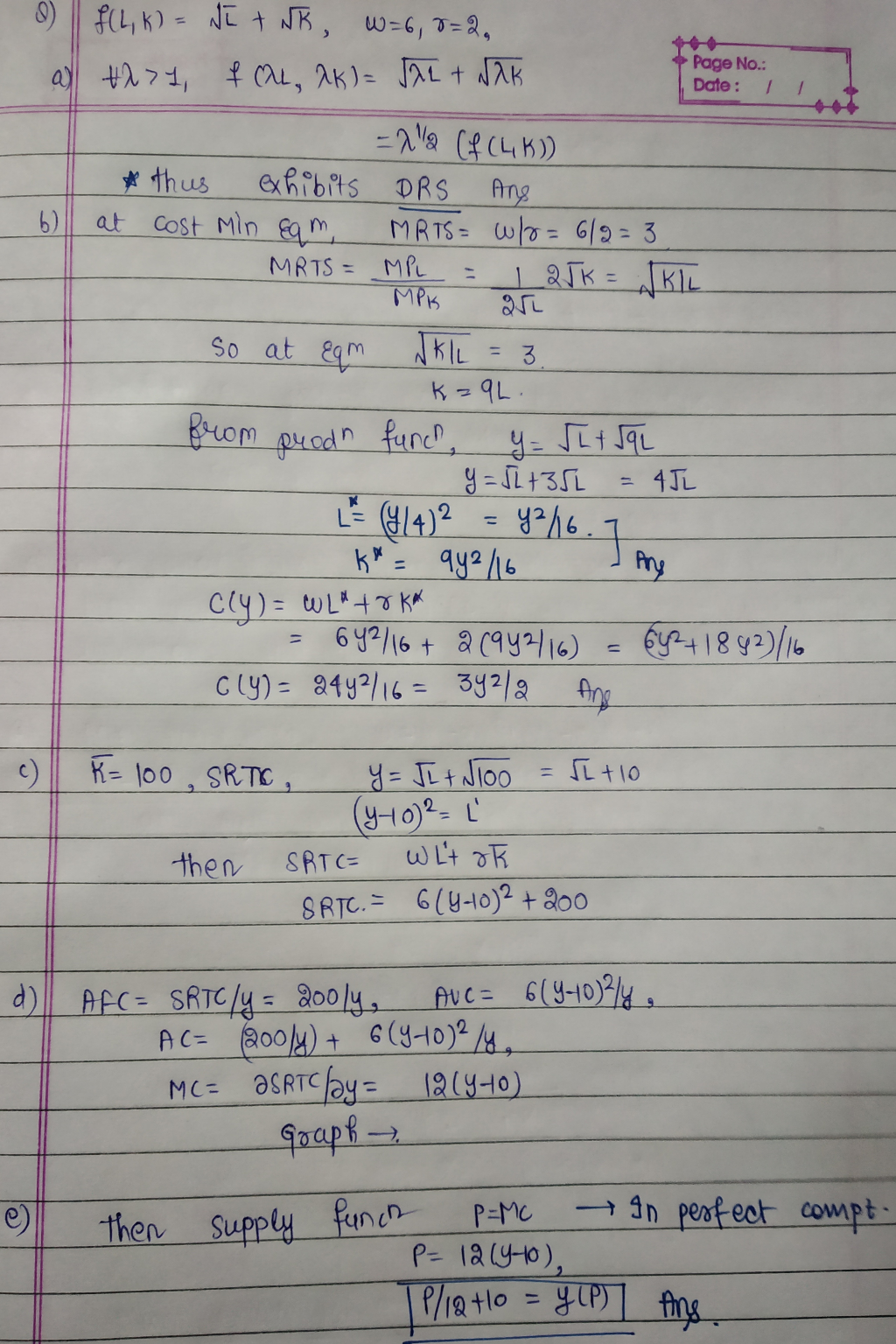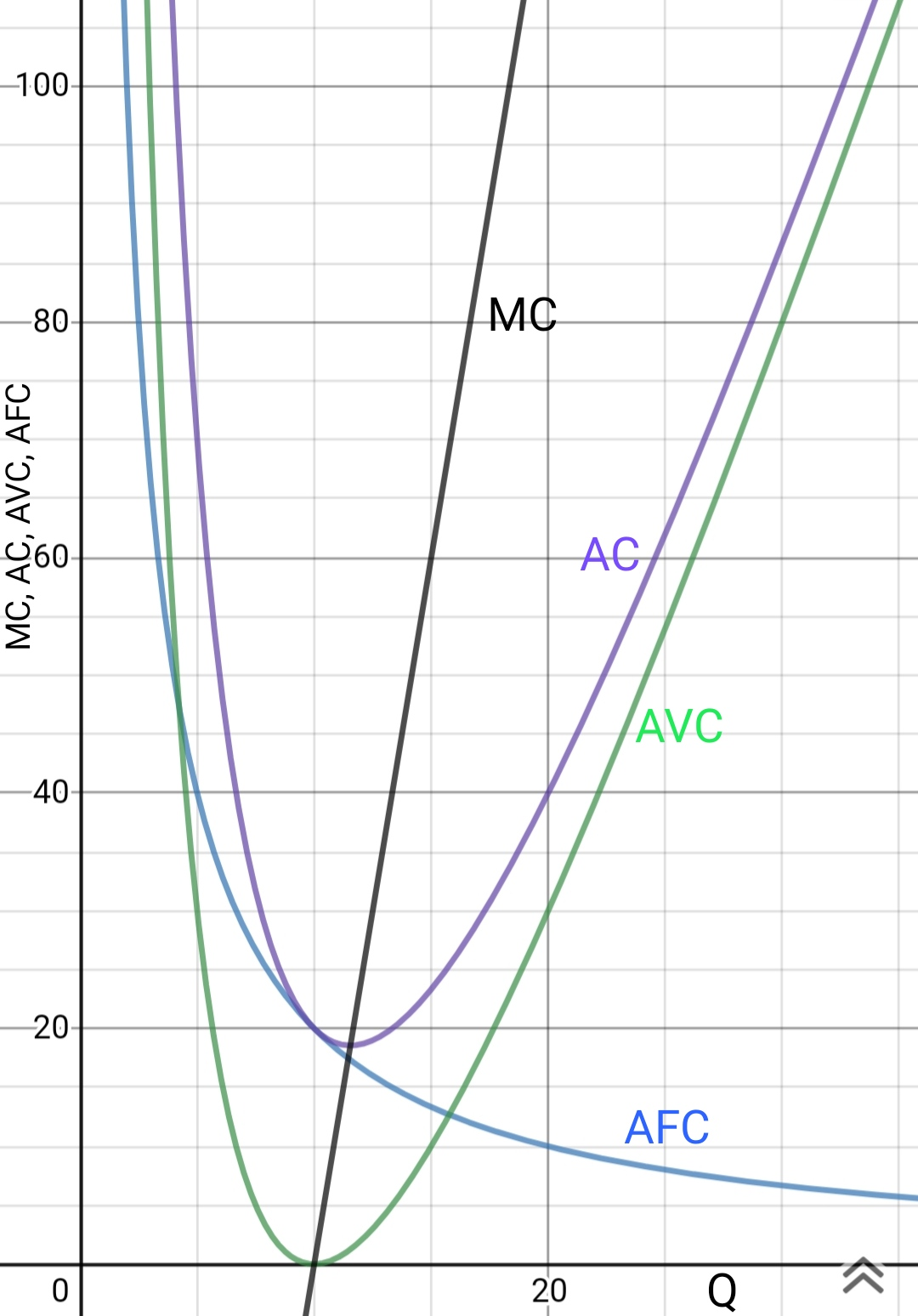In: Economics

# A firm produces output y using two factors of production (inputs), labour L and capital K....

A firm produces output y using two factors of production (inputs), labour L and capital K. The firm’s production function is ?(?,?)=√?+√?=?12+?12. The wage rate w = 6 and the rental price of capital r = 2 are taken as parameters (fixed) by the firm. a. Show whether this firm’s technology exhibits decreasing, constant, or increasing returns to scale. b. Solve the firm’s long run cost minimization problem (minimize long run costs subject to the output constraint) to derive this firm’s i. demand function for labour L = L(y) ii. demand function for capital K = K(y) iii. long run total cost function C = C(y). c. Suppose in the short run, capital is fixed at K = 100. Derive the firm’s short run total cost function C = C(y). d. Derive the AFC, AVC, AC, and MC curves for the firm and graph them on the same diagram – be sure to label them. (Recall: these are short run cost curves). e. Let p be the price of the output y. Derive this firm’s short run supply function y = y(p) assuming it is a competitive firm?

## Solutions

##### Expert SolutionGraph## Related Solutions

##### 3. A perfectly competitive firm produces output y using two factors of production (inputs), labour L...
3. A perfectly competitive firm produces output y using two factors of production (inputs), labour L and capital K. The firm’s production function is ?(?, ?) = (?^1/2 + ?^1/2) ^2. The wage rate is w = 9 and the rental price of capital is r = 1. a. Find the long run equilibrium price p in this market. b. Suppose in the short run, capital is fixed at K = 1. The output price in the short run is...
##### A firm produces output using capital (K) and labor (L). Capital and labor are perfect complements...
A firm produces output using capital (K) and labor (L). Capital and labor are perfect complements and 1 unit of capital is used with 2 units of labor to produce 1 unit of output. Draw an example of an isoquant. If wages and rent are $2 and$3, respectively, what is the Average Total Cost? A firm has a production function given by Q=4KL where K, L and Q denote capital, labor, and output, respectively. The firm wants to produce...
##### A firm uses two inputs in production: capital and labour. In the short run, the firm...
A firm uses two inputs in production: capital and labour. In the short run, the firm cannot adjust the amount of capital it is using, but it can adjust the size of its workforce. What happens to the firm’s average-total-cost curve, the average-variable-cost curve, and the marginal-cost curve when the cost of renting capital increases? The average-total-cost curve (shifts up/shifts down/do not change) . The average-variable-cost curve   (shifts up/shifts down/do not change)  . The marginal-cost curve  (shifts up/shifts down/do not change)   ....
##### A competitive firm uses two inputs, capital (?) and labour (?), to produce one output, (?)....
A competitive firm uses two inputs, capital (?) and labour (?), to produce one output, (?). The price of capital, ??, is $1 per unit and the price of labor, ?? , is$1 per unit. The firm operates in competitive markets for outputs and inputs, so takes the prices as given. The production function is ?(?, ?) = 3? 0.25? 0.25. The maximum amount of output produced for a given amount of inputs is ? = ?(?, ?) units....
##### Consider the following production function using capital (K) and labor (L) as inputs. Y = 10.K0.5L0.5....
Consider the following production function using capital (K) and labor (L) as inputs. Y = 10.K0.5L0.5. The marginal product of labor is (MPL=) 5.K0.5/L0.5, and marginal product of capital (MPK) = 5.L0.5/K0.5.a. If K = 100 and L=100 what is the level of output Y?b. If labor increases to 110 while K=100, what is the level of output?c. If labor increases to 110 while K=100, what is the marginal product of labor?d. If labor increases to 120 while K=100, what...
##### Consider a firm for which production depends on two normal inputs, labour and capital, with prices...
Consider a firm for which production depends on two normal inputs, labour and capital, with prices w and r, respectively. Initially the firm faces market prices of w = 6 and r = 4. The price of capital (r) then shifts to r = 6 while w remains the same. Use isocost-isoquant analysis to show and explain the following. A. In which direction will the substitution effect change the firm’s employment and capital stock? B. In which direction will the...
##### Consider the production function: Y = AK1/3L2/3 . Y is output, K is capital, and L...
Consider the production function: Y = AK1/3L2/3 . Y is output, K is capital, and L is labor. The marginal product of labor is: 2/3A(K/L)1/3 The marginal product of capital is: 1/3A(L/K)2/3 a) Explain how a tax cut on capital promoting capital accumulation can benefit workers. b) Labor unions have often opposed immigration. Using the above equations, explain why. c) Why is productivity growth so important in raising living standards across the population?
##### Consider a producer making choices over two inputs, labour (l) and capital (k) with prices w...
Consider a producer making choices over two inputs, labour (l) and capital (k) with prices w = 3 and r = 1. The production technology is f(l, k) = l + 3k. What is the marginal rate of technical substitution (MRTS)? Is there diminishing MRTS? Find the input demands in long-run (as a function of output level)? Find the long-run total cost, marginal cost, and average cost functions? Do the properties of a typical cost function hold for the long...
##### Suppose your firm uses 2 inputs to produce its output: K (capital) and L (labor). the...
Suppose your firm uses 2 inputs to produce its output: K (capital) and L (labor). the production function is q = 50K^(1/2)L^(1/2). prices of capital and labor are given as r = 2 and w = 8 a) does the production function display increasing, constant, or decreasing returns to scale? how do you know and what does this mean? b) draw the isoquants for your firms production function using L for the x axis and K for y. how are...
##### There are two kinds of factors of production, labor L and capital K, which are only...
There are two kinds of factors of production, labor L and capital K, which are only available in non-negative quantities. There are two ﬁrms that make phones, Apple and Banana. To make qA phones, Apple’s input requirement of (L,K) is given by production function f(L,K) = L0.6K0.2. To make qB phones, Banana’s input requirement of (L,K) is given by production function g(L,K) = L0.75K0.25. (a) (Time: 3 minutes) How many phones can Apple make with factor bundle (L1,K1) = (1,1)?...